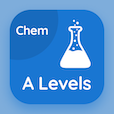Engineering Online Courses

Electromagnetic Theory Quizzes

Electromagnetic Theory Quiz PDF - Complete

# Electromagnetic Theory Multiple Choice Questions p. 47

Study Electromagnetic Theory multiple choice questions and answers, electromagnetic theory quiz answers PDF 47 to study Electromagnetic Theory course online. Electrical Properties of Matter MCQ trivia questions, Electromagnetic Theory Multiple Choice Questions (MCQ) for online college degrees. "Electromagnetic Theory MCQ" PDF eBook: drude-lorentz model, euclidean plane, finite difference time domain history, dielectric permittivity, electromagnetic theory test prep for questions to ask during an interview.

"If substrate is composed of two or more different elements, it is" MCQ PDF: complex, compound, atom, and electron for high school entrance exam. Learn electrical properties of matter questions and answers to improve problem solving skills for online high school college acceptance.

## Electromagnetic Theory Questions and Answers MCQs

MCQ: If substrate is composed of two or more different elements, it is

compound
complex
atom
electron

MCQ: In a free space, E relationship with D is

double of permitivity
inversely proportional
directly proportional
no relationship exist

MCQ: The novelty of Kane Yee's FDTD scheme, presented in his seminal

1900 paper
1944 paper
1966 paper
1988 paper

MCQ: Plane which have set of points satisfying certain relationships, expressible in terms of distance and angle is known as

Euclidean Plane
Dihedral plane
one dimensional plane
zero plane

MCQ: The simplest analysis of the Drude model assumes that electric field E is

non uniform
uniform
infinite
random

### More Quizzes from Electromagnetic Theory Course